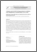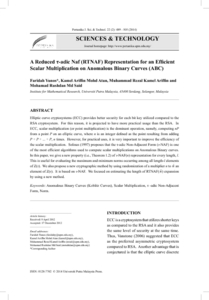# A reduced τ-adic Naf (RTNAF) representation for an efficient scalar multiplication on Anomalous Binary Curves (ABC)

## Citation

Yunos, Faridah and Mohd Atan, Kamel Ariffin and Kamel Ariffin, Muhammad Rezal and Md. Said, Mohamad Rushdan (2014) A reduced τ-adic Naf (RTNAF) representation for an efficient scalar multiplication on Anomalous Binary Curves (ABC). Pertanika Journal of Science & Technology, 22 (2). pp. 489-505. ISSN 0128-7680; ESSN: 2231-8526

## Abstract

Elliptic curve cryptosystems (ECC) provides better security for each bit key utilized compared to the RSA cryptosystem. For this reason, it is projected to have more practical usage than the RSA. In ECC, scalar multiplication (or point multiplication) is the dominant operation, namely, computing nP from a point P on an elliptic curve, where n is an integer defined as the point resulting from adding P + P + ... + P , n times. However, for practical uses, it is very important to improve the efficiency of the scalar multiplication. Solinas (1997) proposes that the τ-adic Non-Adjacent Form (τ-NAF) is one of the most efficient algorithms used to compute scalar multiplications on Anomalous Binary curves. In this paper, we give a new property (i.e., Theorem 1.2) of τ-NAF(n) representation for every length, l. This is useful for evaluating the maximum and minimum norms occurring among all length-l elements of Z(τ). We also propose a new cryptographic method by using randomization of a multiplier n to ñ an element of Z(τ). It is based on τ-NAF. We focused on estimating the length of RTNAF(ñ) expansion by using a new method.Preview
PDF
36248.pdfView Item